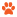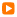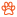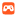Prepdog.org |      | &nb sp; 1st Grade COMMON CORE STATE STANDARDS ENGLISH MATH ADVERTISEMENTLanguage        Conventions of Standard English    L.1.1.B  Use common, proper, and possessive nouns.   L.1.1.B  Use common, proper, and possessive nouns.          Test 3    L.1.1.C  Use singular and plural nouns with matching verbs in basic sentences.  L.1.1.C  Use singular and plural nouns with matching verbs in basic sentences.          Test 5    L.1.1.D  Use personal, possessive, and indefinite pronouns. L.1.1.D  Use personal, possessive, and indefinite pronouns.L.1.1.E  Use verbs to convey a sense of past, present, and future.L.1.1.F  Use frequently occurring adjectives.  L.1.1.G  Use frequently occurring conjunctions.L.1.1.G  Use frequently occurring conjunctions.L.1.1.H  Use determiners.L.1.1.H  Use determiners.L.1.1.I   Use frequently occurring prepositions.          Test 14   L.1.1.I   Use frequently occurring prepositions.L.1.1.J  Produce and expand complete simple and compound declarative, interrogative, imperative, and exclamatory sentences in response to prompts.L.1.2  Demonstrate command of the conventions of standard English capitalization, punctuation, and spelling when writing.  L.1.2.A  Capitalize dates and names of people.L.1.2.A  Capitalize dates and names of people. L.1.2.B  Use end punctuation for sentences.  L.1.2.B  Use end punctuation for sentences. L.1.2.C  Use commas in dates and to separate single words in a series.          Test 22   L.1.2.C  Use commas in dates and to separate single words in a series. L.1.2.C  Use commas in dates and to separate single words in a series. L.1.2.D  Use conventional spelling for words with common spelling patterns and for frequently occurring irregular words.  L.1.2.D  Use conventional spelling for words with common spelling patterns and for frequently occurring irregular words.Operations & Algebraic Thinking       Represent and solve problems involving addition and           subtraction  1.OA.A.1  Use addition and subtraction within 20 to solve word problems1.OA.A.2  Solve word problems that call for addition of three whole numbers whose sum is less than or equal to 20  1.OA.A.1 Use addition and subtraction within 20 to solve word problems 1.OA.A.2  Solve word problems that call for addition of three whole numbers whose sum is less than or equal to 20         Test 5   1.OA.A.2  Solve word problems that call for addition of three whole numbers whose sum is less than or equal to 20       Understand and apply properties of operations and the          relationship between addition and subtraction 1.OA.B.3  Apply properties of operations as strategies to add and subtract.  1.OA.B.3  Apply properties of operations as strategies to add and subtract. 1.OA.B.4  Understand subtraction as an unknown-addend problem. 1.OA.B.4  Understand subtraction as an unknown-addend problem.         Test 10  1.OA.B.4  Understand subtraction as an unknown-addend problem.      Add and subtract within 20 1.OA.C.5  Relate counting to addition and subtraction  1.OA.C.5  Relate counting to addition and subtraction      Work with addition and subtraction equations 1.OA.D.7  Understand the meaning of the equal sign, and determine if equations involving addition and subtraction are true or false.         Test 14   1.OA.D.7  Understand the meaning of the equal sign, and determine if equations involving addition and subtraction are true or false. 1.OA.D.8  Determine the unknown whole number in an addition or subtraction equation relating three whole numbers.         Test 16   1.OA.D.8  Determine the unknown whole number in an addition or subtraction equation relating three whole numbers.Number & Operations in Base Ten       Extend the counting sequence 1.NBT.A.1  Count to 120 1.NBT.A.1  Count to 120       Understand place value  1.NBT.B.2.A  10 can be thought of as a bundle of ten ones — called a "ten." 1.NBT.B.2.A  10 can be thought of as a bundle of ten ones — called a "ten." 1.NBT.B.2.B  The numbers from 11 to 19 are composed of a ten and one, two, three, four, five, six, seven, eight, or nine ones.         Test 6   1.NBT.B.2.B  The numbers from 11 to 19 are composed of a ten and one, two, three, four, five, six, seven, eight, or nine ones. 1.NBT.B.2.C  The numbers 10, 20, 30, 40, 50, 60, 70, 80, 90 refer to one, two, three, four, five, six, seven, eight, or nine tens (and 0 ones).         Test 8   1.NBT.B.2.C  The numbers 10, 20, 30, 40, 50, 60, 70, 80, 90 refer to one, two, three, four, five, six, seven, eight, or nine tens (and 0 ones). 1.NBT.B.3  Compare two two-digit numbers based on meanings of the tens and ones digits, recording the results of comparisons with the symbols >, =, and <.         Test 10   1.NBT.B.3  Compare two two-digit numbers based on meanings of the tens and ones digits, recording the results of comparisons with the symbols >, =, and <.      Use place value understanding and properties of        operations to add and subtract1.NBT.C.4  Add within 1001.BNT.C.5  Given a two-digit number, mentally find 10 more or 10 less than the number, without having to count; explain the reasoning used.         Test 13   1.BNT.C.5  Given a two-digit number, mentally find 10 more or 10 less than the number, without having to count; explain the reasoning used. 1.NBT.C.6  Subtract multiples of 10 in the range 10-90 from multiples of 10 in the range 10-90 (positive or zero differences)Measurement & Data       Measure length indirectly and by iterating length units 1.MD.A.1  Order three objects by length; compare the lengths of two objects indirectly by using a third object.         Test 2   1.MD.A.1  Order three objects by length; compare the lengths of two objects indirectly by using a third object. 1.MD.A.2  Express the length of an object as a whole number of length units, by laying multiple copies of a shorter object (the length unit) end to end         Test 4   1.MD.A.2  Express the length of an object as a whole number of length units, by laying multiple copies of a shorter object (the length unit) end to end       Tell and write time 1.MD.B.3  Tell and write time in hours and half-hours using analog and digital clocks.       Represent and interpret data1.MD.C.4  Organize, represent, and interpret data with up to three categories         Test 7   1.MD.C.4  Organize, represent, and interpret data with up to three categoriesGeometry       Reason with shapes and their attributes         Test 1   1.G.A.1  Distinguish between defining attributes         Test 2   1.G.A.1  Distinguish between defining attributes 1.G.A.3  Partition circles and rectangles into two and four equal shares         Test 4   1.G.A.3  Partition circles and rectangles into two and four equal shares         Test 5   1.G.A.3  Partition circles and rectangles into two and four equal shares ADVERTISEMENT The Prepdog volunteers are working hard to transfer new tests.  As this is a mind numbing operation, we will upload the tests as a "Beta" release of sorts.  We ask your help for another eye to correct typos and mind numbing mistakes.  If you find any mistakes please click this link and include the URL, the question, and answer with the issue.  Otherwise we hope to go through all the questions as soon as possible.  If you demand perfection please donate a significant amount of funds and we will hire an editor, otherwise we thank you for your patience and we hope that the 19+ years of 24/7 time spent on Prepdog has provided help to students and teachers. Copyright © 2009- 2015 Prepdog.org, All Rights Reserved Common Core State Standards © Copyright 2010. National Governors Association Center for Best Practices and Council of Chief State School Officers. All rights reserved.